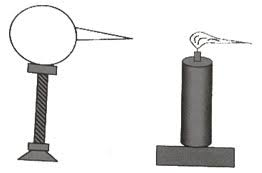# ElectrostaticsElectric charge

Charge is the property of matter that causes it to produce and experience electrical and magnetic effects. The study of the electrical charges at rest is called electrostatics.

There are two types of charges in nature : positive and negativeLike charges repel, and unlike charges attract, each other.

The excess or deficiency of electrons in a body gives the concept of charge. If there is an excess of electrons in a body, it is negatively charged. And if there is deficiency of electrons, the body becomes positively charged. Whenever addition or removal of electrons takes places, the body acquires a charge.

The SI Unit of charge is coulomb (C).It is scalar quantity.

Properties of Charge

1.Quantization of Charge :  Electric charge can have only discrete values, rather than any value. That is, charge is quantized. The smallest discrete value of charge that can exist in nature is the charge on an electron, given as  , e =  ± 1.6 x 10- 19 C

A charge q must be an integral multiple of this basic unit. That is,

Q = ± ne          where n = 1, 2, …

Charge on a body can never be (½)e, (2/3)e, or 5.7e, etc.

2.Conservation of Charge : In an isolated system, the total charge remains constant. In other words, charge can neither be created nor destroyed. It can be transferred from one body to the other. Or, equal amounts of positive or negative charges can appear or disappear.

3.charge is associated with mass  : when the body is given positive charges,the mass of body decreases and when the body is given positive charges, the mass of body decreases.

4.The magnitude of charge on a body is not affected by the speed of the body.

Charging by Induction

When charging a conductor by induction, a charged object is brought close to but does not touch the conductor. In the end the conductor has charge of the opposite sign as the charge on the object.

One way to carry out the four-step process:

1.    Bring the charged object close to, but not touching, the conductor. Charge on the conductor shifts in response to the nearby charged object.

2.   Connect the conductor to ground. Ground is basically a charge reservoir - anything that can give up or receive charge without noticing the change. Electrons flow from ground to the conductor if the charged object is positive, and the opposite way if the object is negative. The conductor now has a net charge with a sign opposite to the sign on the charged object.

3.    Remove the ground connection. The transferred electrons can't get back to where they came from.

4.    Remove the charged object. The net charge distributes itself over the surface of the conductor.CHARGING BY CONDUCTION

Charging by conduction involves the contact of a charged object to a neutral object.  Hence when an uncharged conductor is brought in contact with a charged conductor, charge is shared between the two conductors and hence the uncharged conductor gets charged.
During charging by conduction, both objects acquire the same type of charge.Surface Density of charge

The surface density of charge is defined as the amount of charge per unit area of a charged surface. It is denoted by σ.

Formula:-

Surface density(σ)=Q/A

Here, Q is the charge distributed uniformly over the surface area (A) of a conductor.

Action of points

The wind may made to blow a candle flame aside as shown in the figure. Each particle moves away, carries with it a small amount of charge from the conductor. The action of losing charged conductor due to its pointed ends is called action of points. The action of points is used in lightning in conductors.